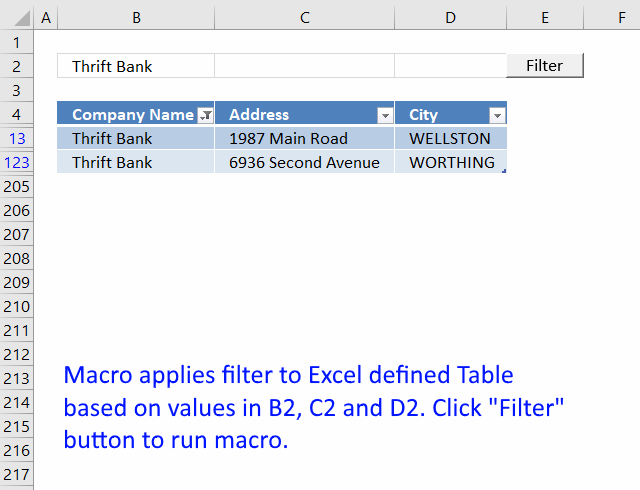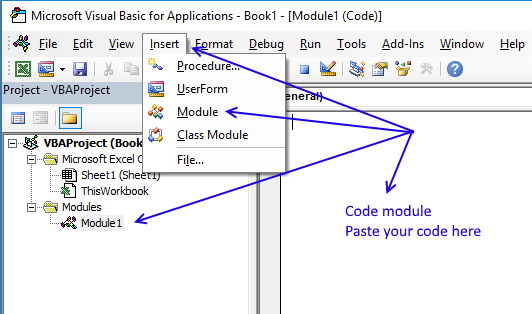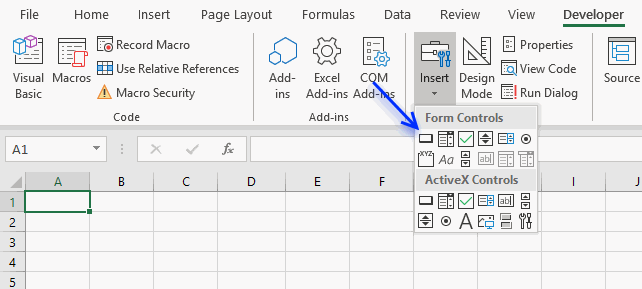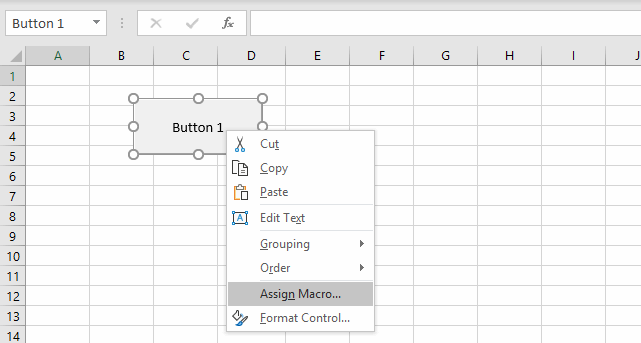Author: Oscar Cronquist Article last updated on February 01, 2019In this tutorial, I am going to demonstrate how to filter an Excel define Table through a VBA macro.

### How it works

1. Type a value in cell range B2, C2 or D2.
2. Press Filter button

The table column below is instantly filtered. If the cell value is empty, no filter is applied.

### VBA code

```Sub TblFilter()

If Worksheets("Sheet1").Range("A2") <> "" Then
Worksheets("Sheet1").ListObjects("Table1").Range.AutoFilter _
Field:=1, Criteria1:="=" & Worksheets("Sheet1").Range("A2")
Else
ActiveSheet.ListObjects("Table1").Range.AutoFilter Field:=1
End If

If Worksheets("Sheet1").Range("B2") <> "" Then
Worksheets("Sheet1").ListObjects("Table1").Range.AutoFilter _
Field:=2, Criteria1:="=" & Worksheets("Sheet1").Range("B2")
Else
ActiveSheet.ListObjects("Table1").Range.AutoFilter Field:=2
End If

If Worksheets("Sheet1").Range("C2") <> "" Then
Worksheets("Sheet1").ListObjects("Table1").Range.AutoFilter _
Field:=3, Criteria1:="=" & Worksheets("Sheet1").Range("C2")
Else
ActiveSheet.ListObjects("Table1").Range.AutoFilter Field:=3
End If

End Sub```

### Where to copy vba code?1. Copy above VBA macro (CTRL + c)
2. Press Alt+F11 to open the Visual Basic Editor.
3. Press with left mouse button on "Insert" on the menu.
4. Press with left mouse button on "Module" to create a module.
5. Paste code to module (CTRL + v)
6. Exit VBE and return to Excel.

### How to create a button

1. Press with left mouse button on "Developer" tab.
2. Press with left mouse button on "Insert" button.
3. Create a button (Form Control).4. Press with left mouse button on and drag on your worksheet to create the button.
5. Press with right mouse button on on your new button.6. Select a macro to assign.
7. Press with left mouse button on OK button.

The assigned macro is rund when the user press with left mouse button ons on the button.

### Get the Excel fileDynamic-filtering-excel-tables.xlsm